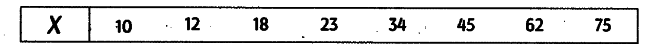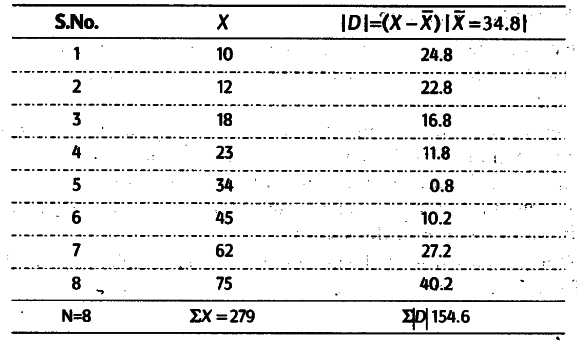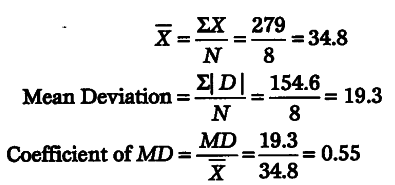# Find the mean deviation and coefficient of mean deviation?

Find the mean deviation and coefficient of mean deviation from the following dataCalculation of Mean Deviation from Arithmetic Mean and Coefficient of Mean Deviation